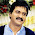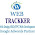## Friday, 23 August 2013

### Symmetric Key Encryption / Decryption using KeyTool

There are three ways for encryption/decryption using symmetric key in java.
1. Generating Symmetric key using Cryptop Library pro-grammatically. Refer Java Symmetric Key Encryption Crypto Library
2. Generate Symmetric Key using Key Tool. Current blog.
3. Use plain text as a symmetric key. Refer Java Symmetric Key Encryption using KeyTool

In this blog we will generate the symmetric key using Java KeyTool program. For more information on Key Tool read blog Java Key tool Tutorial. And then we will encrypt the data using this symmetric key.

To understand the concepts of symmetric/asymmetric key encryption read blog Java Security.

Below video explains the mechanism of symmetric key Encryption

Generate Keystore with symmetric key

```keytool -genseckey -alias mykey -keyalg AES -keysize 128 -storetype jceks -keystore mykeystore.jks
```

The above command will create a keystore with a private key called mykey. Both keystore and private key can be accessed using a password. Below diagram illustrates the same.

The above command uses AES algorithm to generate the symmetric key. There are other algorithm as well like DES (56), DESede (168), HmacSHA1, HmacSHA256.
AES is Advanced Encryption System algorithm. The data will be encrypted using this Algorithm. The key size mentioned in 128, so the generated key will be of 128 bit. The key size can be 128,192,256 according to the AES specification.
Store type used is jceks which stands for Java Cryptography Extension. The other store type that can be used is jks which is Java Key Store.

For a maven project you will need the apache-common-codec jar as a dependency or else just download the jar and paste it in the folder which is in the classpath.

Maven dependency:
```<dependency>
<groupId>commons-codec</groupId>
<artifactId>commons-codec</artifactId>
<version>1.8</version>
</dependency>
```

Also place the above generated symmetric key at the root folder of the project.

Java Code

```import java.io.FileInputStream;
import java.io.UnsupportedEncodingException;
import java.security.InvalidKeyException;
import java.security.Key;
import java.security.KeyStore;
import java.security.NoSuchAlgorithmException;

import javax.crypto.Cipher;
import javax.crypto.IllegalBlockSizeException;
import javax.crypto.SecretKey;
import javax.crypto.spec.SecretKeySpec;

import org.apache.commons.codec.binary.Base64;

public class SymmetricKeyWithKeyTool {
public static void main(String[] args) {
try {
KeyStore keyStore = KeyStore.getInstance("JCEKS");
FileInputStream stream = new FileInputStream("mykeystore.jks");

String data="ABC";

//Encrypt Data
String encryptedData = encryptWithAESKey(data, key.getEncoded());

System.out.println("Encrypted Data : " + encryptedData);

//Decrypt Data
System.out.println("Decrypted Data : " +decryptWithAESKey(encryptedData, key.getEncoded()));

} catch (Exception e) {
// TODO Auto-generated catch block
e.printStackTrace();
}

}

public static String encryptWithAESKey(String data, byte[] key) throws NoSuchAlgorithmException, NoSuchPaddingException,
SecretKey secKey = new SecretKeySpec(key, "AES");

Cipher cipher = Cipher.getInstance("AES");

cipher.init(Cipher.ENCRYPT_MODE, secKey);
byte[] newData = cipher.doFinal(data.getBytes());

return Base64.encodeBase64String(newData);
}

public static String decryptWithAESKey(String inputData, byte[] key) throws NoSuchAlgorithmException,
Cipher cipher = Cipher.getInstance("AES");
SecretKey secKey = new SecretKeySpec(key, "AES");

cipher.init(Cipher.DECRYPT_MODE, secKey);
byte[] newData = cipher.doFinal(Base64.decodeBase64(inputData.getBytes()));
return new String(newData);

}
}

```

Output
```Encrypted Data : zavs8vg2y6iqsr+9budWuw==
Decrypted Data : ABC
```

You can get the source code from

For more on Encryption/Decryption, read below blogs.
• Without Keytool

1.2.3.4.5.6.7.